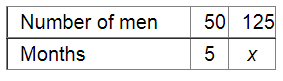# A work force of 50 men with a contractor can finish a piece of work in 5 months.`
Question:

A work force of 50 men with a contractor can finish a piece of work in 5 months. In how many months the same work can be completed by 125 men?

Solution:

Let x be the number days required to complete a piece of work by 125 men.Since the number of men engaged and the number of days taken to do a piece of work are in inverse variation, we have:

$50 \times 5=125 x$

$\Rightarrow x=\frac{50 \times 5}{125}$

$=2$

Thus, the required number of months is 2 .